## Eccentricity

A quantity defined for a Conic Section which can be given in terms of Semimajor and Semiminor Axes for an Ellipse. For an Ellipse with Semimajor Axisand Semiminor Axis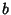,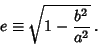The eccentricity can be interpreted as the fraction of the distance to the semimajor axis at which the Focus lies,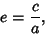where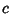is the distance from the center of the Conic Section to the Focus. The table below gives the type of Conic Section corresponding to various ranges of eccentricity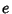.Curve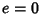Circle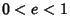Ellipse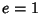Parabola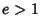Hyperbola

See also Circle, Conic Section, Eccentric Anomaly, Ellipse, Flattening, Hyperbola, Oblateness, Parabola, Semimajor Axis, Semiminor Axis